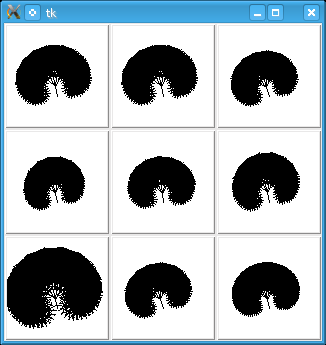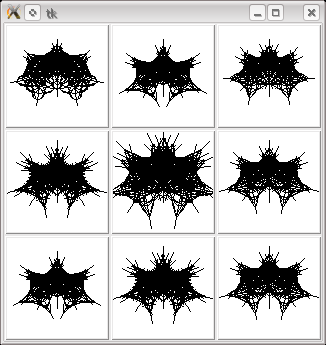# Evolving The Blind Watchmaker

Recently I’ve been reading the classic book by Richard Dawkins, The Blind Watchmaker. In it he begins by discussing how evolution can produce complex systems from only a few simple rules. He demonstrates this using a simple tree drawing algorithm in which a few ‘genes’ control aspects such as the number of branches and the branch angle. The trees are evolved solely through mutation of an initial tree, rather combing the ‘genes’ of two trees to produce a child, and introducing mutations in those children.

In reality evolution is driven by pressures from the environment on the genes and those that produce the fittest host will survive. As this is early in the book though Dawkins uses himself as the environment and manually picks the most visually appealing trees.

Although the book is essentially timeless as although new evidence is continually being found in favour of evolution, the general thrust remains true. The passages where he talks about his computer, however, have dated horribly (which is not surprising given it was first published in 1986!). In this post I’ll describe how to recreate the section where he describes evolving trees in Python so you can create your own trees on your pc.

As with the book our trees will be controlled by nine genes, each of which is an integer. Dawkins doesn’t state what the nine genes do as for his purposes that would confuse matters, but for us it’s vital. Fortunately figure three allows us to work out what genes one, five, seven and nine do for ourselves.

• Horizontal scaling
• Number of branches per level
• Length of first branch
• Scaling factor for length of subsequent branches
• Vertical scaling
• Angle of first branch
• Angle of branching
• Scaling factor for angle of branching
• Levels of branching

The gene descriptions in bold are those that I deduced from the book, the others are ones I decided on myself. The first step in writing a program like this is to decide exactly how these genes will affect the drawn tree. To do this we create a series of functions, one for each gene, that converts the gene value into a value that can be used by the drawing code. These functions are given below.

``````horiz_scaling = lambda dna: (dna+10.0)/10.0
branches = lambda dna: dna
initial_length = lambda dna: dna + 10
length_scaling = lambda dna: (dna+10.0)/10.0
vert_scaling = lambda dna: (dna+10.0)/10.0
initial_angle = lambda dna: dna/10.0
initial_angle_of_branching = lambda dna: 1.0+dna/5.0
change_in_angle_between_branches = lambda dna: dna/5.0
max_levels = lambda dna: dna
``````

These functions are used by the `draw_branch` function which renders a single line, and recursively calls itself to draw the next level of branches.

``````def draw_branch(img, dna, level, start, angle, length, angle_between_all_branches):
end = (start + math.sin(angle) * length * horiz_scaling(dna),
start - math.cos(angle) * length * vert_scaling(dna))
img.line(start + end, (0, 0, 0))
if level >= max_levels(dna):
return
else:
branch_angle = angle - angle_between_all_branches/2.0
angle_between_branches =
0 if branches(dna) == 0 else angle_between_all_branches/branches(dna)
for i in range(branches(dna)+1):
draw_branch(img, dna, level+1, end,
branch_angle + angle_between_branches*i, length*length_scaling(dna),
angle_between_all_branches + change_in_angle_between_branches(dna))
``````

To start the drawing process off we need a function, `draw_tree`, which calls the branch drawing function with the initial values for the length of branch and angle between the subbranches.

``````def draw_tree(img, dna):
draw_branch(img, dna, 0, (50, 70),
initial_angle(dna), initial_length(dna), initial_angle_of_branching(dna))
``````

Now we can draw a tree a we need to be able to generate the children of tree, which we do by picking a gene and either incrementing or decrementing it. A couple of genes make no sense if they are negative so they code prevents these from going below zero.

``````def evolve(dna):
gene = random.choice(range(9))
if (gene in [1, 8] and dna[gene] == 0) or random.random() < 0.5:
dna[gene] += 1
else:
dna[gene] -= 1
return dna
``````

If we combine these functions with a simple TK-based interface, as shown below, we exactly the abilities described in The Blind Watchmaker book. Nine possible trees are displayed, when the user clicks on one nine new children are created and displayed.Without more details about the original program it’s hard to recreate it exactly, but this program is a decent starting point. Happy evolving!

To run this program yourself you’ll need to download and install Python from python.org and the PIL image library. Next code the sourcecode below into a file called “darwkins_trees.py” and double click on it.

``````#!/usr/bin/env python
#
# Redistribution and use in source and binary forms, with or without modification, are
# permitted provided that the following conditions are met:
#
#   1. Redistributions of source code must retain the above copyright notice, this list of
#      conditions and the following disclaimer.
#   2. Redistributions in binary form must reproduce the above copyright notice, this list
#      of conditions and the following disclaimer in the documentation and/or other materials
#      provided with the distribution.
#
# THIS SOFTWARE IS PROVIDED BY <COPYRIGHT HOLDER> ``AS IS'' AND ANY EXPRESS OR IMPLIED
# WARRANTIES, INCLUDING, BUT NOT LIMITED TO, THE IMPLIED WARRANTIES OF MERCHANTABILITY AND
# FITNESS FOR A PARTICULAR PURPOSE ARE DISCLAIMED. IN NO EVENT SHALL <COPYRIGHT HOLDER> OR
# CONTRIBUTORS BE LIABLE FOR ANY DIRECT, INDIRECT, INCIDENTAL, SPECIAL, EXEMPLARY, OR
# CONSEQUENTIAL DAMAGES (INCLUDING, BUT NOT LIMITED TO, PROCUREMENT OF SUBSTITUTE GOODS OR
# SERVICES; LOSS OF USE, DATA, OR PROFITS; OR BUSINESS INTERRUPTION) HOWEVER CAUSED AND ON
# ANY THEORY OF LIABILITY, WHETHER IN CONTRACT, STRICT LIABILITY, OR TORT (INCLUDING
# NEGLIGENCE OR OTHERWISE) ARISING IN ANY WAY OUT OF THE USE OF THIS SOFTWARE, EVEN IF
# ADVISED OF THE POSSIBILITY OF SUCH DAMAGE.
#
# The views and conclusions contained in the software and documentation are those of the
# authors and should not be interpreted as representing official policies, either expressed
# or implied, of Andrew Wilkinson.
import math, os, random, sys
from Tkinter import *
import Image, ImageTk, ImageDraw
image_size = (100, 100)
samples = 9
horiz_scaling = lambda dna: (dna+10.0)/10.0
branches = lambda dna: dna
initial_length = lambda dna: dna + 10
length_scaling = lambda dna: (dna+10.0)/10.0
vert_scaling = lambda dna: (dna+10.0)/10.0
initial_angle = lambda dna: dna/10.0
initial_angle_of_branching = lambda dna: 1.0+dna/5.0
change_in_angle_between_branches = lambda dna: dna/5.0
max_levels = lambda dna: dna
def draw_branch(img, dna, level, start, angle, length, angle_between_all_branches):
end = (start + math.sin(angle) * length * horiz_scaling(dna), start - math.cos(angle) * length * vert_scaling(dna))
img.line(start + end, (0, 0, 0))
if level >= max_levels(dna):
return
else:
branch_angle = angle - angle_between_all_branches/2.0
angle_between_branches = 0 if branches(dna) == 0 else angle_between_all_branches/branches(dna)
for i in range(branches(dna)+1):
draw_branch(img, dna, level+1, end, branch_angle + angle_between_branches*i, length*length_scaling(dna), angle_between_all_branches + change_in_angle_between_branches(dna))
def draw_tree(img, dna):
draw_branch(img, dna, 0, (50, 70), initial_angle(dna), initial_length(dna), initial_angle_of_branching(dna))
def evolve(dna):
gene = random.choice(range(9))
if (gene in [1, 8] and dna[gene] == 0) or random.random() < 0.5:
dna[gene] += 1
else:
dna[gene] -= 1
return dna
class Application(Frame):
def __init__(self, master=None):
Frame.__init__(self, master)
self.dna = [0, 1, 0, 0, 0, 0, 0, 0, 1]
self.grid()
self.create_widgets()
self.create_choices()
def create_widgets(self):
self.buttons = []
for i in range(samples):
button = Button(self)
button["command"] = self.choose_tree(i)
button.grid(row=i / 3, column=i % 3)
self.buttons.append(button)
def create_choices(self):
self.choices = [evolve(self.dna[:]) for i in range(samples)]
self.images = [Image.new("RGB", image_size, (255, 255, 255)) for _ in range(samples)]
[draw_tree(ImageDraw.Draw(self.images[i]), self.choices[i]) for i in range(samples)]
self.tkimages = [ImageTk.PhotoImage(image) for image in self.images]
for i in range(samples):
self.buttons[i]["image"] = self.tkimages[i]
def choose_tree(self, i):
def func():
self.dna = self.choices[i]
self.create_choices()
return func
if __name__ == "__main__":
root = Tk()
app = Application(master=root)
app.mainloop()
root.destroy()
``````

Photo my tree at dusk by b k.